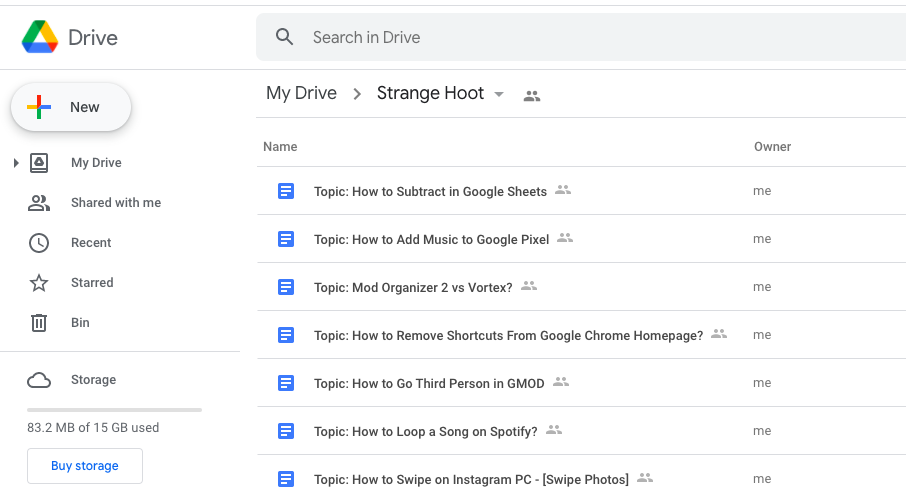# How to Subtract in Google Sheets?

Google sheets is a best product while the users are working on cost sheets or any cost analysis work together via sharing. Financial functions work easily and seamlessly with a team.

Putting formulas in Google Sheets is simple and easy to use for those who are well versed with MS Excel. The only difference is the Google sheets give best experience and simple to use interface working with Formula.

How to Subtract in Google Sheets

### Open Google Sheets with Share option• The system asking you to create and share appears. Do that.
• The Google sheet opens up with the Share button on the top right.
• The subtract function can be used in different scenarios.

### Use Subtract function – Scenario 1

The Sales Manager wants to get the product cost after deducting discounts on products. Here is how he uses the subtract function to determine the value.

In the below sheet, the product is USB stick and its Price is USD 100 and the discount applied on the price is USD 1.15. Calculate the Net price.

1. Make the following columns in the sheet.
• A1 = Product
• B1 = Price
• C1 = Discount
• D1 = Net
2. Put the values in each column.
• A2 = USB Stick
• B2 = 100
• C2 = 1.15
• D2 = use the formula as shown.
• =B2-C2
1. Press ENTER on D2 cell. You shall see the output as below.
1. You have done subtraction successfully.

### Use Subtract function – Scenario 2

John has a dataset in which he needs to put the subtraction formula to obtain values. The dataset consists of daily finances. John wants to match the money in hand at the end of today after spending at different places.

1. Enter date and opening balance (money in hand).
2. Enter the expenses for the daily stuff bought from that money.
3. The dataset is built as below.
1. In C7, put the formula as =C1-SUM(C2:C6). ENTER.
2. We have summed up all expenses and subtracted from the Opening Bal. (C1) value.
1. Simple to use and quick to obtain results.

### Takeaway

Google Sheets is one of the most useful products of Google. Working with formulas with a team on the dataset is the most convenient in Google Sheets. Once the user starts using Google Sheets, he/she cannot go away from the sheets. It gives awesome user experience.